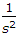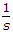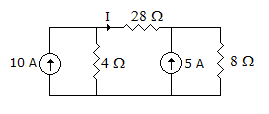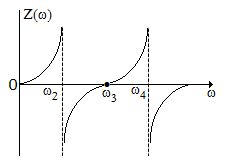# Electronics and Communication Engineering - Networks Analysis and Synthesis

1.

Fourier transform and F(jω) of a signal f(t) has the property

 A. F(jω) = F(-jω) B. F(jω) = -F(-jω) C. F(jω) = F*(jω) D. F(jω) = -F*(-jω)

Explanation:

No answer description available for this question. Let us discuss.

2.

If f(t) = δ(t), F(s) =

 A.B.C. 1 D. s

Explanation:

No answer description available for this question. Let us discuss.

3.

In figure, the current I isA. 1 A B. 2 A C. 4 A D. 8 A

Explanation:

No answer description available for this question. Let us discuss.

4.

Figure shows the variation of Z(ω) for a positive real function. The function isA. RC impedance B. RL impedance C. RLC impedance D. Reactance function

Explanation:

No answer description available for this question. Let us discuss.

5.

The voltage drop per ampere-metre of an aluminium conductor is higher as compared to a copper conductor of the same size due to its higher

 A. insulation resistance B. resistivity C. conductivity D. current density